# The Josephus Problem: Part 3: Solving the Double Step Case

Posted in Computer Science

This is Part 3 of an N-part series.

## Solving the Double Step Case

The Josephus Problem for a step size of $$m = 2$$ can be solved two ways:

• Algorithm D: Doubling Permutation Algorithm
• Algorithm S: Label Skipped Nodes Algorithm

In this blog post we cover Algorithm D, which makes use of a doubling permutation.

In the first section (Algorithm D: Using Doubling Permutation) we start by showing the recipe as applied to the case of $$n = 11, m = 2$$.

The recipe is straightforward to apply, but it is not at all clear how it works. We start by introducing the algorithm, then carrying it out for the case of $$n = 11, m = 2$$ (this is a familiar case that we have covered in prior posts).

We make observations about the procedure by comparing the case of $$n = 8, m = 2$$ (a special case where the circle size is a power of two) before returning to the example of $$n = 11, m = 2$$ to make generalizations and give a shortcut method for the general double-step case.

## Algorithm D: Using Doubling Permutation

In Exercise 29, Knuth asks a question that lays out a solution procedure for the special case of $$m = 2$$, and asks the reader to prove it:

Prove: the cycle form of the Josephus permutation when $$m = 2$$ can be obtained by expressing the "doubling permutation" of $$\{1, 2, \dots, 2n\}$$, which takes $$j$$ into $$(2j) \mod (2n+1)$$ into cycle form, then reversing L and R, and erasing all numbers larger than $$n$$.

Fortunately, we don't have to work out the solution procedure for ourselves; unfortunately, it is not at all obvious why the solution procedure works, so once we have seen how to apply it, we have to do a bit more work before we can understand it.

### The Algorithm

Given an input size $$n$$, the algorithm steps are:

1. Write the doubling permutation (in table form)

2. Factor the doubling permutation into cycles

3. Reverse each cycle and remove values larger than n

The result will be the Josephus permutation cycles.

### Write the Doubling Permutation

As mentioned above, we will start with the concrete example of $$n = 11, m = 2$$.

We start by writing the doubling permutation for the integers $$1 \dots 2n$$:

$$\left( 1 \, 2 \, 4 \, 8 \, 16 \, 32 \, \dots \right)$$

When a number is greater than $$2n+1$$, it is reduced $$\mod (2n+1)$$, so the next few terms of the doubling permutation written out and reduced modulo $$2n+1$$ are:

$$\left( 1 \, 2 \, 4 \, 8 \, 16 \, 32 \, 64 \, 128 \, 256 \, 512 \, 1024 \right)$$

which reduces modulo $$2n+1$$ to:

$$\left( 1 \, 2 \, 4 \, 8 \, 16 \, 9 \, 18 \, 13 \, 3 \, 6\, 12 \right)$$

After 12, we reach 1 again, the starting value, so further doublings will result in repetition of the elements we have so far.

Next, continue the process with the remaining elements. Start with the smallest element not included in the cycle found above, which is 5, and continue the operation of doubling and reducing modulo $$2n+1$$. Doing this results in the terms:

$$\left( 5 \, 10 \, 20 \, 40 \, 80 \, \dots \right)$$

which reduces modulo $$2n+1$$ to:

$$\left( 5 \, 10 \, 20 \, 17 \, 11 \, \dots \right)$$

Repeating this until the first element repeats yields all of the remaining elements:

$$\left( 5 \, 10 \, 20 \, 17 \, 11 \, 22 \, 21 \, 19 \, 15 \, 7 \, 14 \right)$$

Now the final doubling permutation can be written as the product of the two cycles:

$$\left( 1 \, 2 \, 4 \, 8 \, 16 \, 9 \, 18 \, 13 \, 3 \, 6\, 12 \right) \left( 5 \, 10 \, 20 \, 17 \, 11 \, 22 \, 21 \, 19 \, 15 \, 7 \, 14 \right)$$

We now have the cycles of the doubling permutation! Step 2 finished.

### Table Method

Slightly more convenient than writing out the cycles the way we did above is to create a table, with a column for $$j = 1 \dots 2n$$, a column for $$2j$$, and a column for $$(2j) \mod (2n+1)$$.

 j   |  2j  |  2j mod 2n+1
-----|------|---------------
1  |   2  |        2
2  |   4  |        4
3  |   6  |        6
4  |   8  |        8
5  |  10  |       10
6  |  12  |       12
7  |  14  |       14
8  |  16  |       16
9  |  18  |       18
10  |  20  |       20
11  |  22  |       22
12  |  24  |        1
13  |  26  |        3
14  |  28  |        5
15  |  30  |        7
16  |  32  |        9
17  |  34  |       11
18  |  36  |       13
19  |  38  |       15
20  |  40  |       17
21  |  42  |       19
22  |  44  |       21


Now the cycles can be constructed by starting with the $$j$$ column of the first row in the table (1), reading off the corresponding entry (2) in the $$(2j) \mod (2n+1)$$ column, adding the pair $$1 \rightarrow 2$$ to the cycle, and moving to the row of the table with that corresponding $$j$$ value.

The next step takes us to row 2 of the table, where we read off the value 4 from the right column, add the pair $$2 \rightarrow 4$$ to the cycle, and keep moving.

Eventually, the procedure will complete the first cycle and take us back to 1. Since there are still rows in the table left, repeat the procedure, starting with the first available row of the table (5), and keep going until all entries of the table are gone. This will yield the same cycles as the process described above, but the bookkeeping is slightly easier:

$$\left( 1 \, 2 \, 4 \, 8 \, 16 \, 9 \, 18 \, 13 \, 3 \, 6\, 12 \right) \left( 5 \, 10 \, 20 \, 17 \, 11 \, 22 \, 21 \, 19 \, 15 \, 7 \, 14 \right)$$

### Reverse the Doubling Permutation

The next step is to reverse the permutation from left to right, which means we step through all cycles from left to right, and step through each cycle from left to right.

Starting with the doubling permutation:

$$\left( 1 \, 2 \, 4 \, 8 \, 16 \, 9 \, 18 \, 13 \, 3 \, 6\, 12 \right) \left( 5 \, 10 \, 20 \, 17 \, 11 \, 22 \, 21 \, 19 \, 15 \, 7 \, 14 \right)$$

We obtain the reverse:

$$\left( 14 \, 7 \, 15 \, 19 \, 21 \, 22 \, 11 \, 17 \, 20 \, 10 \, 5 \right) \left( 12 \, 6 \, 3 \, 13 \, 18 \, 9 \, 16 \, 8 \, 4 \, 2 \, 1 \right)$$

### Trim the Reversed Doubling Permutation

Now we eliminate any numbers from the reversed doubling permutation that are larger than $$n = 11$$, to get the trimmed permutation:

$$\left( 7 \, 11 \, 10 \, 5 \right) \left( 6 \, 3 \, 9 \, 8 \, 4 \, 2 \, 1 \right)$$

This is the final Josephus permutation. The one remaining step is to rewrite the cycles in standard "sorted" order:

$$\left( 1 \, 6 \, 3 \, 9 \, 8 \, 4 \, 2 \right) \left( 5 \, 7 \, 11 \, 10 \right)$$

Congratulations! You just solved the problem.

## Why Does Algorithm D Work?

If at this point you are scratching your head in wonder at the seeming black magic involved in this algorithm, then welcome to The Art of Computer Programming! (Seriously, the exercises are chock full of questions like this one.)

To understand why Algorithm D works, it is instructive to consider a case where $$n$$ is a power of two, and make some observations and generalizations to uncover the pattern at work in Algorithm D.

### An Important Observation

Start with an important observation: each time we complete one circuit of the circle, we have reduced the number of items in the circle by

$$\left\lfloor{ \frac{n}{2} }\right\rfloor$$

If our circle size is even, that means we cut our circle size in half each time through the circle.

The halving operation means the Josephus problem is intimately linked with powers of 2 (or, base 2 logs), so that's where the powers of 2 will come from.

### Three Facts

Now we use this observation about halving to state 3 facts about the case:

Fact 1: If we are considering an item at an odd-numbered location in the circle, we have already been through the circle at least once.

(Explanation: The first time through the circle, all of the items in the circle at even-numbered locations will be removed, so only items at odd-numbered locations around the circle are left after the first pass.)

Fact 2: When the circle size is a power of 2, the first item in the circle is the last item removed.

(Explanation: If we have a circle the size of a power of 2, we will always remove the last item in the circle when we complete a circuit (because we will always reach it using two-steps), which means we always skip item 1 if the circle size is a power of 2.)

(Addendum: If a circle size starts as a power of 2, going through the circle once and removing elements will exactly halve the circle size, and preserve the property that the circle size is a power of 2.)

Fact 3: When the circle size is not a power of 2, the size will eventually be reduced to a power of 2. Then the item at the starting position in that round will be the last item removed.

(Explanation: Combining Fact 1 and Fact 2, we can see that eventually we will always reach the special case of the circle size being a power of 2, since with each step, we reduce the circle size by 1. For example, for a circle size of 22, we must carry out 6 removal operations to reach a circle size of 16, at which point the item at the starting position will be the last item to be removed from the circle.)

Now we will use these 3 facts to understand Algorithm D.

### Power of Two Example

Let's run through a Josephus solution for an example where $$n$$ is a power of two, specifically, $$n = 8, m = 2$$.

We start by creating the doubling permutation table, with columns for $$j$$, $$2j$$, and $$(2j) \mod (2n+1)$$:

 j   |  2j  |  2j mod 2n+1
-----|------|---------------
1  |   2  |        2
2  |   4  |        4
3  |   6  |        6
4  |   8  |        8
5  |  10  |       10
6  |  12  |       12
7  |  14  |       14
8  |  16  |       16
9  |  18  |        1
10  |  20  |        3
11  |  22  |        5
12  |  24  |        7
13  |  26  |        9
14  |  28  |       11
15  |  30  |       13
16  |  32  |       15


The variable $$j$$ takes on $$2n$$ values, $$j = 1 \dots 2n$$, each of which are doubled and reduced modulo $$2n+1$$.

From column 3 we can see why the doubling permutation requires us to consider $$2n$$ items instead of $$n$$ items: because the first $$n$$ items are always even (cuz, uh, we're doubling), and what we want is coverage of odds and evens in the range $$1 \dots n$$. This is also why we reduce $$\mod (2n + 1)$$, which is guaranteed to be odd - if we reduced $$\mod 2n$$ we would run into the same problem of wrapping back garound to the even integers.

If we step through the doubling permutation to factor it into its cycles, we start at 1 and connect it to 2. This states that item 2 is removed 1st, so we write 1 -> 2 for the doubling permutation cycle, and keep in mind that it will be reversed to 2 -> 1 when we write the final Josephus permutation cycle.

The next step is to move to 2, and connect it to 4, so 2 -> 4 (also recognizing that this means the opposite connection 4 -> 2, that item 4 is removed second, in the final Josephus permutation.)

Now we would expect to see 3 -> 6, since we know that item 6 is removed 3rd with the same certainty that we know item 4 is removed 2nd. But the problem is that it doesn't fit into the existing cycle, so it may belong later in the cycle or in another cycle altogether. We can't be certain.

This is why we multiply our step size by 2 each time (2, 4, 8, 16), instead of incrementing it by 2 each time (2, 4, 6, 8, 10).

Fact 1 stated that any items at even-numbered positions are eliminated on the first pass - we can see that manifest as we write the doubling cycle. Once we reach 16 (double the size of the circle), the integers begin to loop back around through the odd numbers (also thanks to the choice of the odd modulus $$2n+1$$):

$$\left( 1 \, 2 \, 4 \, 8 \ 16 \, 15 \, 13 \, 9 \right)$$

Consider the $$16 \rightarrow 15$$ connection. Stepping through the (doubled) circle the first time through, we end on 16. The next doubling takes us 16 more steps forward, but that's 1 less than the size of the circle (again due to the choice of an odd modulus $$2n+1$$), so we end up at $$2n - 1$$. 15 is larger than the size of the circle, so it will be excluded in the final Josephus cycle.

Continuing to follow this through, we reach 9, which will also be excluded from the Josephus cycle, and 9 returns back to 1 and completes the cycle.

Now we continue the process with the remaining items in the table, starting with the smallest, which is 3. Doubling 3, we see the 3 -> 6 connection we mentioned earlier, which we expected to see since it indicates the known fact that item 6 will be removed 3rd.

$$\left( 3 \, 6 \, 12 \, 17 \, 14 \, 11 \, 5 \, 10 \right)$$

Finally, reversing and trimming the values larger than 8:

$$\left( 5 \, 7 \, 6 \, 3 \right) \left( 8 \, 4 \, 2 \, 1 \right)$$

and writing in sorted order:

$$\left( 1 \, 8 \, 4 \, 2 \right) \left( 3 \, 5 \, 7 \, 6 \right)$$

Fact 2 tells us that the item in position 1 is the last to be removed, since the size of the circle $$n = 8 = 2^3$$.
We confirm the cycle does contain $$1 \rightarrow 8$$ (meaning, the item in position 1 will be removed eighth).

### Not a Power of Two Example

Now that we've looked at an example where the size of the circle is a power of 2, let's return to the original example that we presented in Part 2 and the beginning of this blog post, namely, $$n = 11, m = 2$$.

Here is the doubling permutation table again:

 j   |  2j  |  2j mod 2n+1
-----|------|---------------
1  |   2  |        2
2  |   4  |        4
3  |   6  |        6
4  |   8  |        8
5  |  10  |       10
6  |  12  |       12
7  |  14  |       14
8  |  16  |       16
9  |  18  |       18
10  |  20  |       20
11  |  22  |       22
12  |  24  |        1
13  |  26  |        3
14  |  28  |        5
15  |  30  |        7
16  |  32  |        9
17  |  34  |       11
18  |  36  |       13
19  |  38  |       15
20  |  40  |       17
21  |  42  |       19
22  |  44  |       21


As before, the last column splits into odd and even, with all the evens coming first, so we consider $$2n$$ elements to cover all $$n$$ odd and even items, and use an odd modulus to ensure we don't wrap back around to the even numbers the second time through.

We follow the entries of the table to factor this permutation into cycles, as above, yielding the two cycles:

$$\left( 1 \, 2 \, 4 \, 8 \, 16 \, 9 \, 18 \, 13 \, 3 \, 6 \, 12 \right)$$

and

$$\left( 5 \, 10 \, 20 \, 17 \, 11 \, 22 \, 21 \, 19 \, 15 \, 7 \, 14 \right)$$

Finally, we reverse these and eliminate the numbers larger than $$n$$, giving the Josephus permutation cycle:

$$\left( 6 \, 3 \, 9 \, 8 \, 4 \, 2 \, 1 \right) \left( 7 \, 11 \, 10 \, 5 \right)$$

or, rearranging into sorted order:

$$\left( 1\, 6 \, 3 \, 9 \, 8 \, 4 \, 2 \right) \left( 5 \, 7 \, 11 \, 10 \right)$$

However, there is a faster way to figure out the last item in the circle that will be removed that only requires us to carry out 3 steps.

### The Power of Two Shortcut

Unlike the circle whose size was a power of two, we don't know ahead of time who goes last. However, Fact 3 tells us that eventually our circle will reach the size of a power of two. For this case it only takes 3 steps (11 - 8). At that point, the circle item at the starting point for the next step will be the last item removed.

Here is the diagram for the third removal operation (left labels show removal indices, right labels show circle position indices):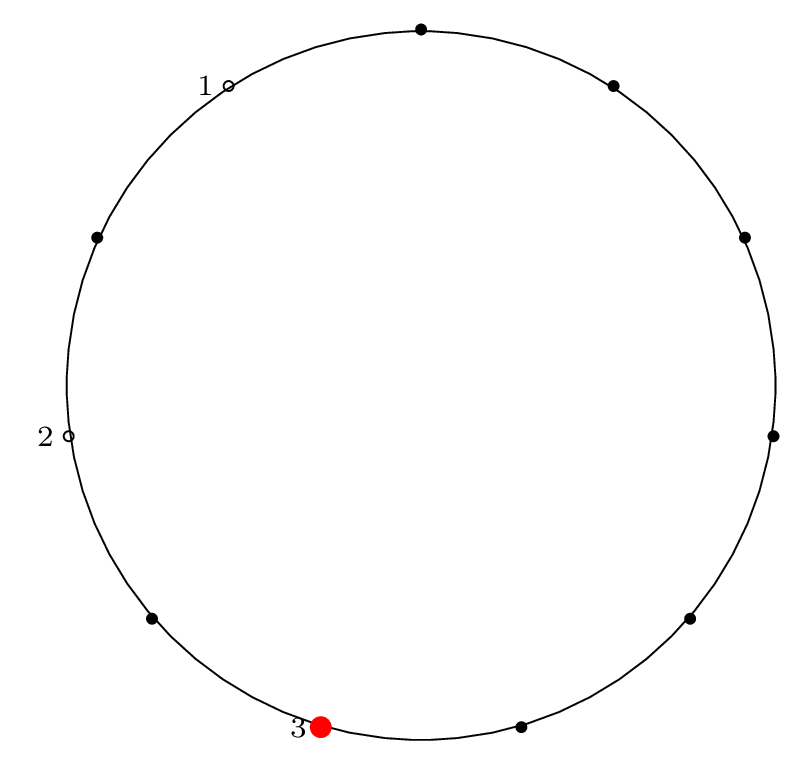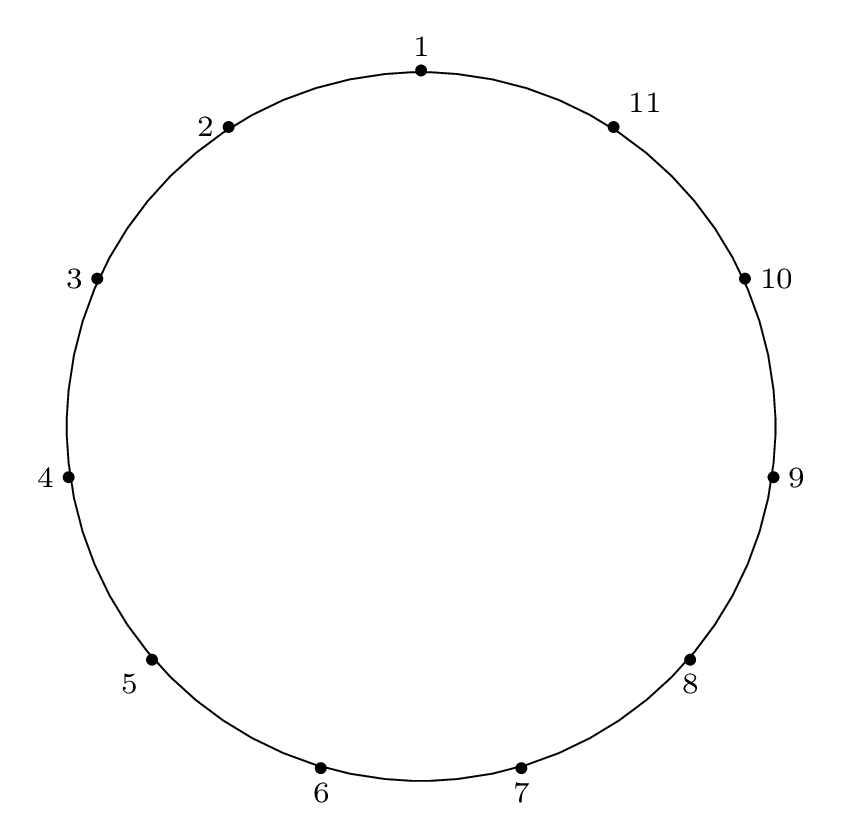Fact 3 is in effect here, because after the third item is removed, our starting "cursor" is on item 7 in the circle, and the circle is of size $$2^3$$, so from Fact 2 we know that 7 will be the last item removed from the circle.

We can see from the final permutation that $$7 \rightarrow 11$$, so indeed, this holds true.

To translate this into mathematical terms, we are carrying out $$r$$ operations, where

$$r = n - 2^{ \left\lfloor{ \lg{n} }\right\rfloor }$$

and at the conclusion of those $$r$$ operations, we mark the starting item for the next round. That item will be the last item to be removed.

## Algorithm D Summary

To summarize what we covered in this blog post:

This post covers a method for solving the Josephus problem for the special case of a step size of two, $$m = 2$$.

We started by covering the recipe for Algorithm D, which uses a doubling permutation to obtain the Josephus permutation in cycle form. We illustrated the three steps of the recipe (populate the table, write the doubling permutation cycles, then filter and reverse) using an example case.

Then we got into the question of why Algorithm D works, by making an observation and building upon it. We deduced several facts that allowed us to come up with a shortcut for the $$m = 2$$ case.

Our final solution technique (making use of the above-mentioned shortcut) is as follows:

1. Carry out as many removal operations as needed to make the circle the size of a power of 2 (see formula for $$r$$ above)

2. The starting item for the next round (where our "removal cursor" will start counting) will be the last item removed from the circle.

So if you find yourself being rounded up in some sort of weird mass execution scheme that happens to be a Josephus problem with a step size of 2, the key to surviving is to remember your powers of 2, to carry out $$r = n - 2^{ \left\lfloor{ \lg{n} }\right\rfloor }$$ removal operations, and to grab the next open spot in the circle.

# The Josephus Problem: Part 2: Two Examples

Posted in Computer Science

This is Part 2 of an N-part series.

## Two Examples

In this blog post we'll walk through two examples of a Josephus problem:

$$n = 8, m = 4$$

and

$$n = 11, m = 2$$

We will simulate the Josephus process and show how the solution is expressed using both permutation notation and cycle notation.

## n = 8, m = 4

We begin with the example that Knuth gives in his problem statement, $$n = 8, m = 4$$, along with its solution, $$54613872$$.

Here is the Josephus circle for this case, and the corresponding removal index on the right (the red dot indicates the starting point for the process):

 Circle index: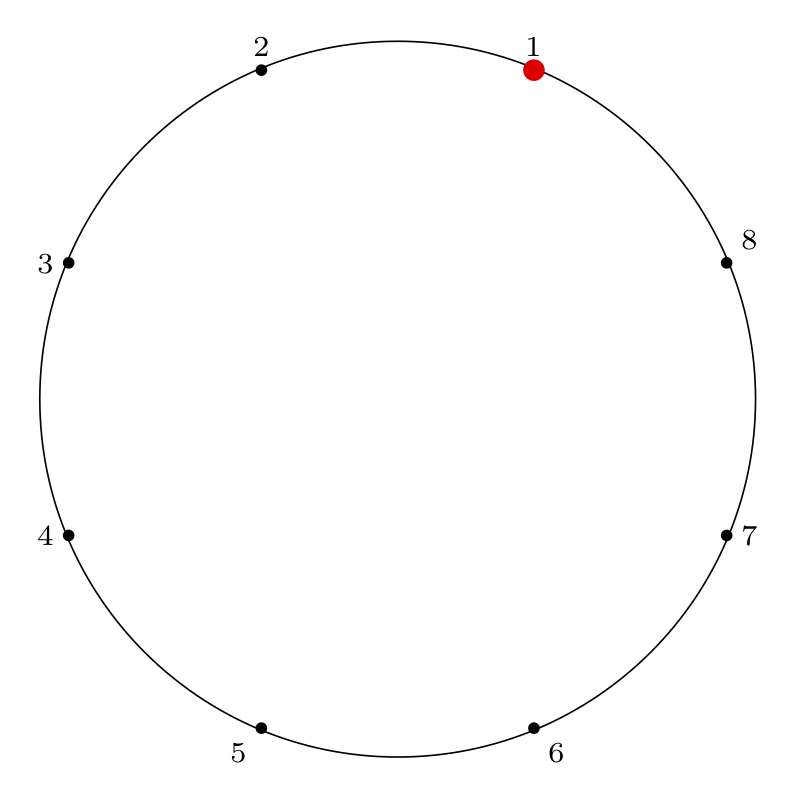Removal index: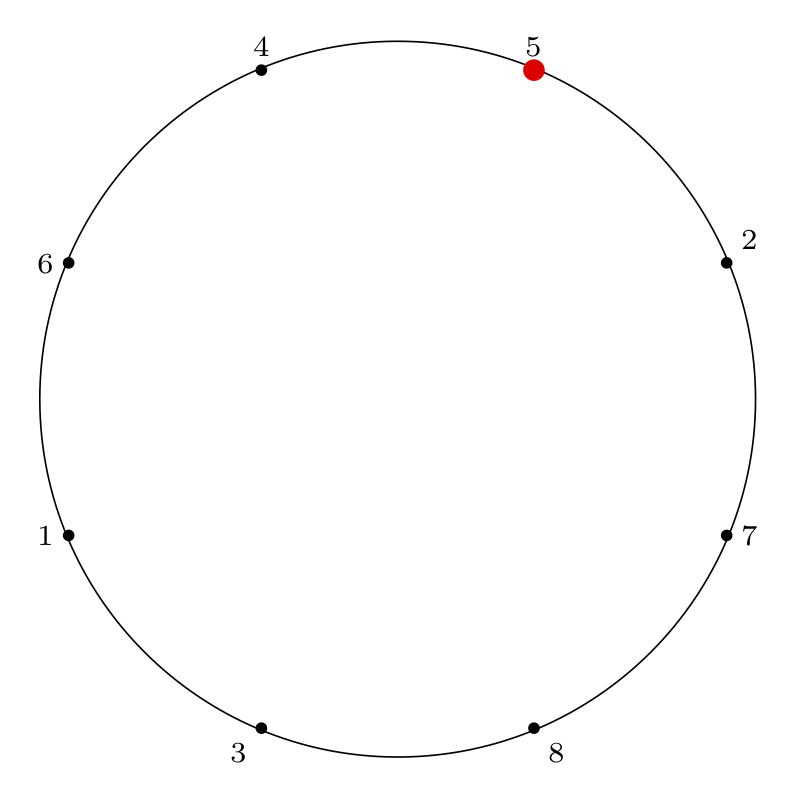### Step by Step Removal for n = 8

Starting at the red dot, we proceed $$m-1$$ points forward through the circle ($$m$$ if we include the starting point), skipping items that have already been removed, and remove an item from the circle.

We indicate the starting point with a red dot in Step 0, then indicate the removal index with a red dot and the corresponding removal index.

 Step 0: Starting Point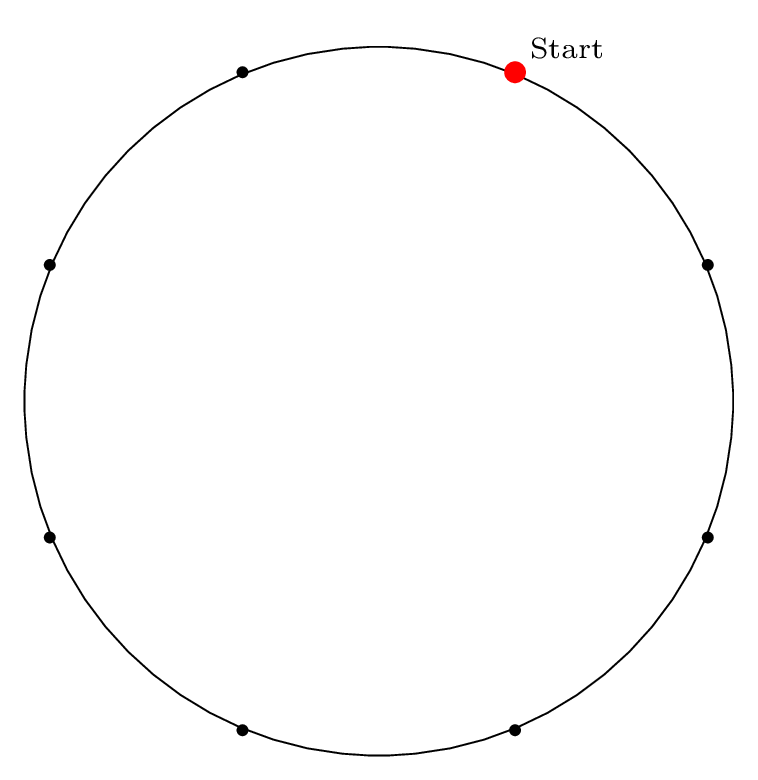Step 1: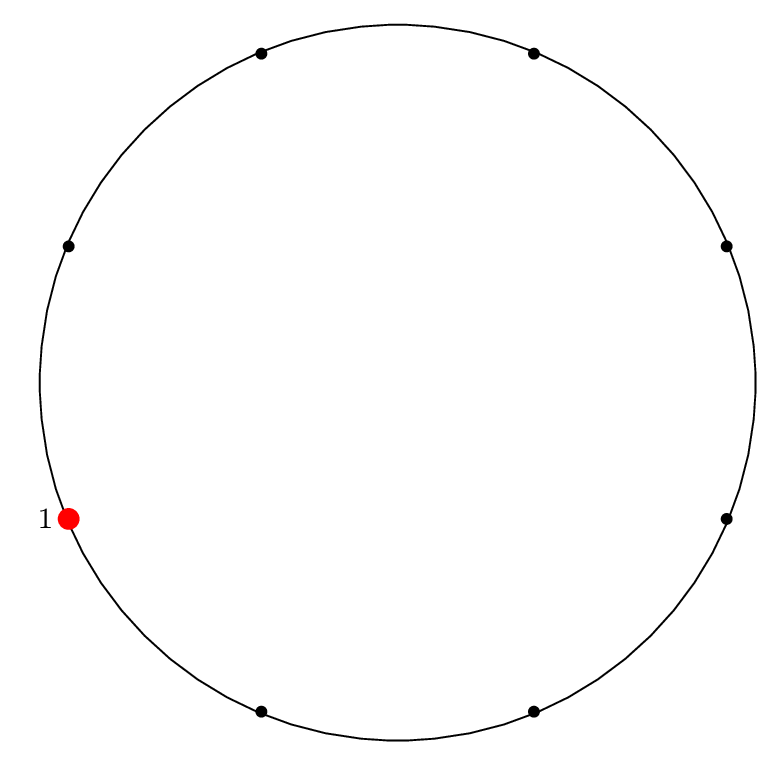Step 2: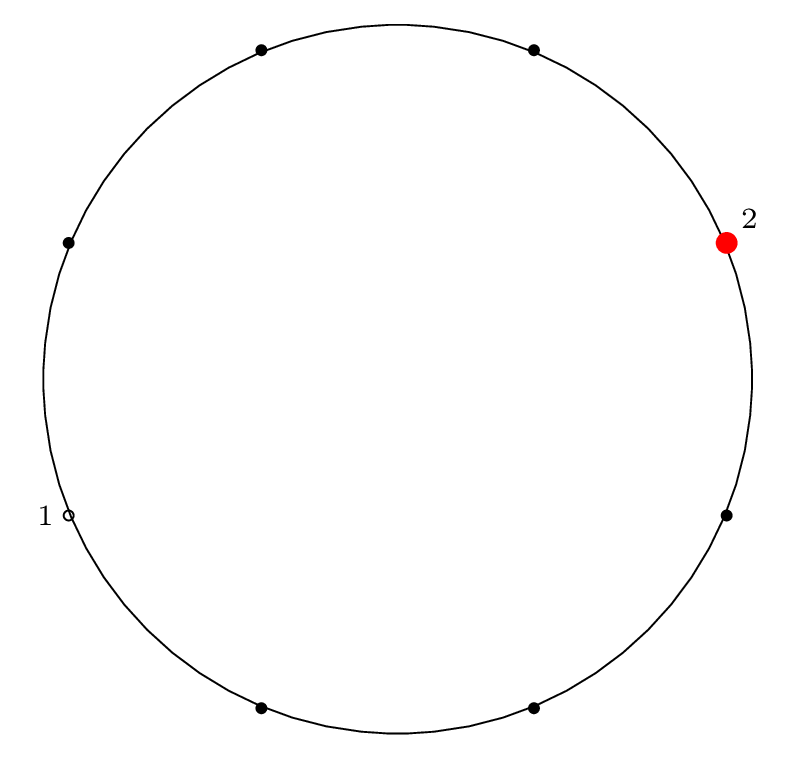Step 3: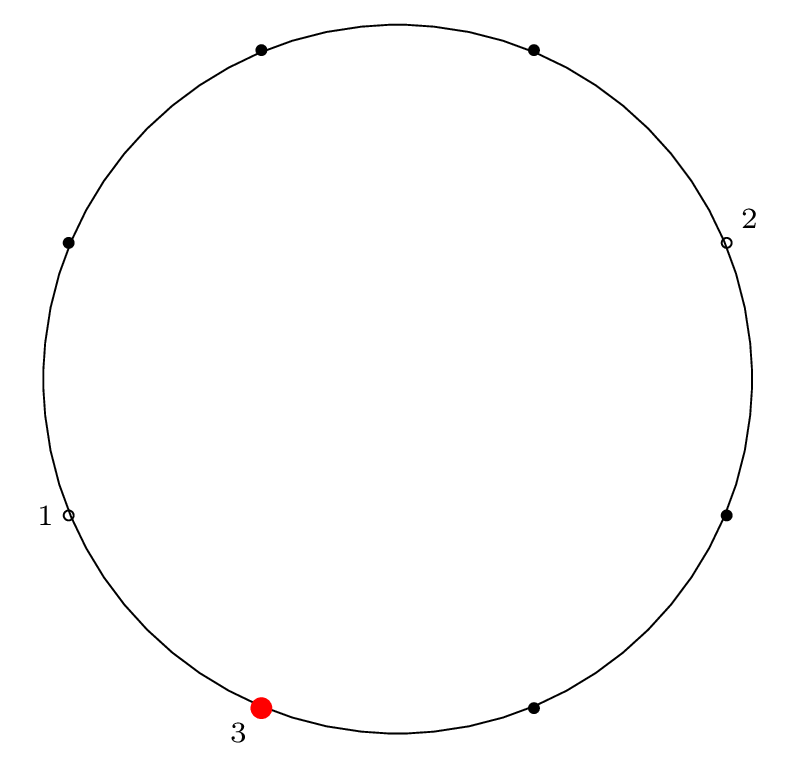Step 4: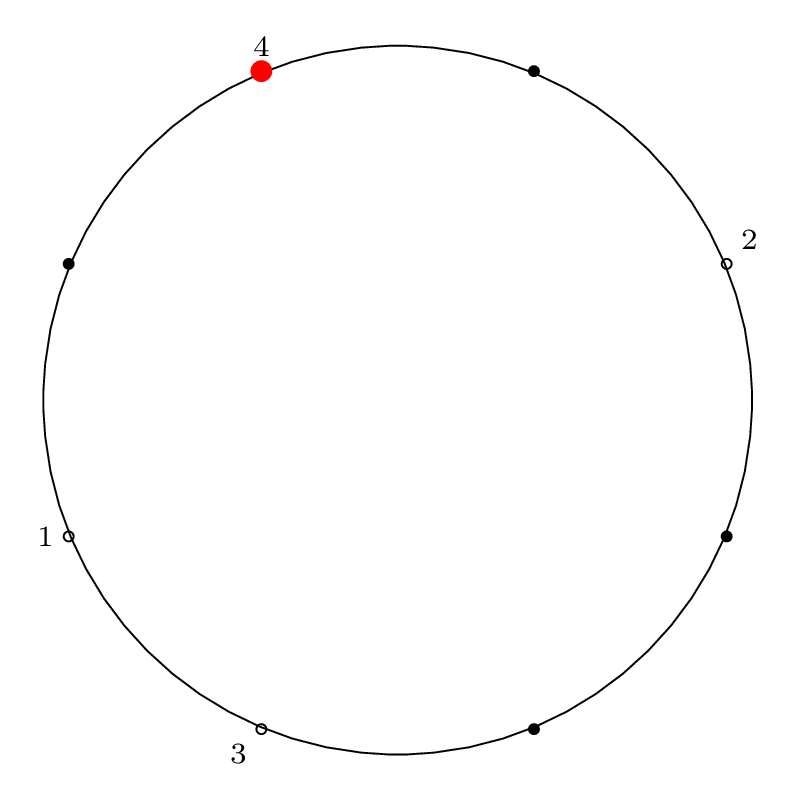Step 5: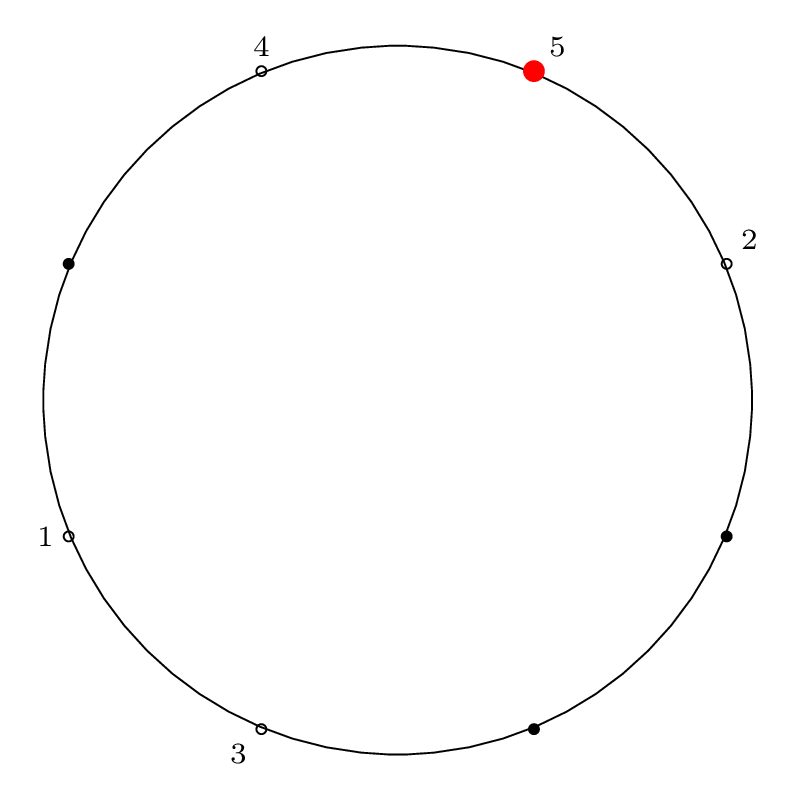Step 6: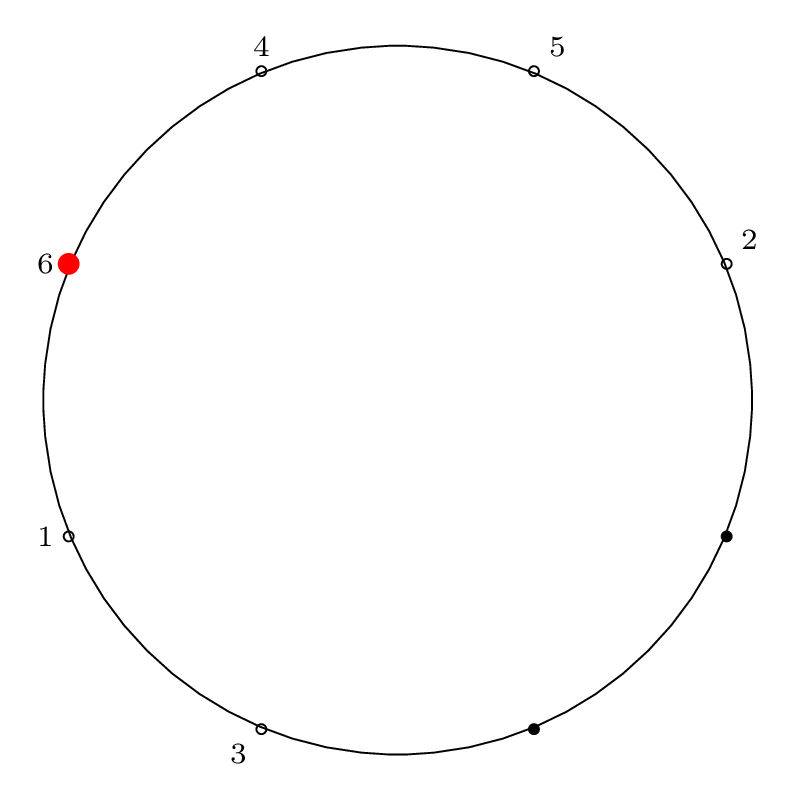Step 7: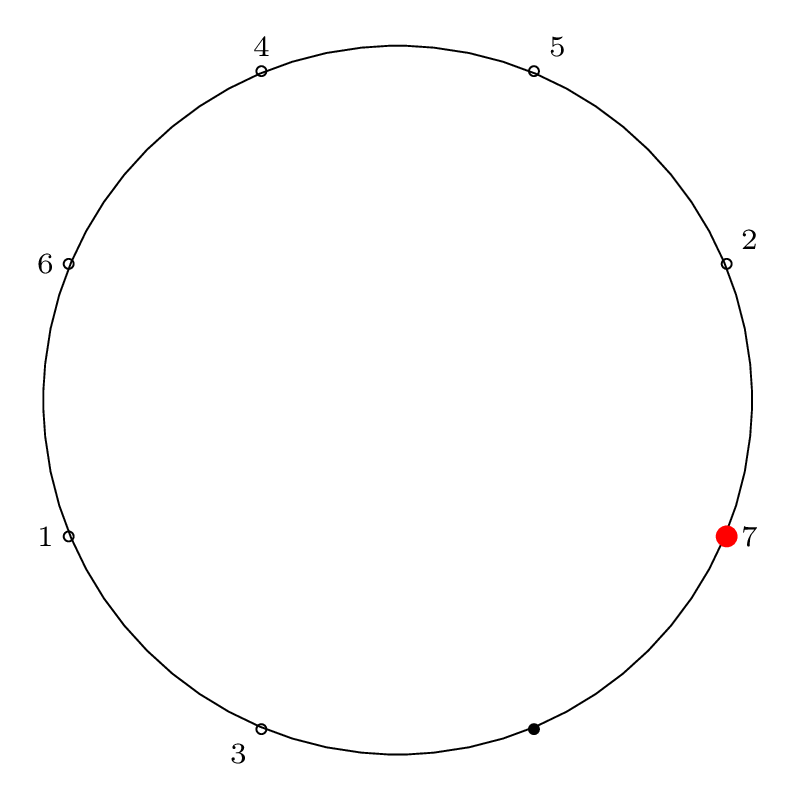Step 8: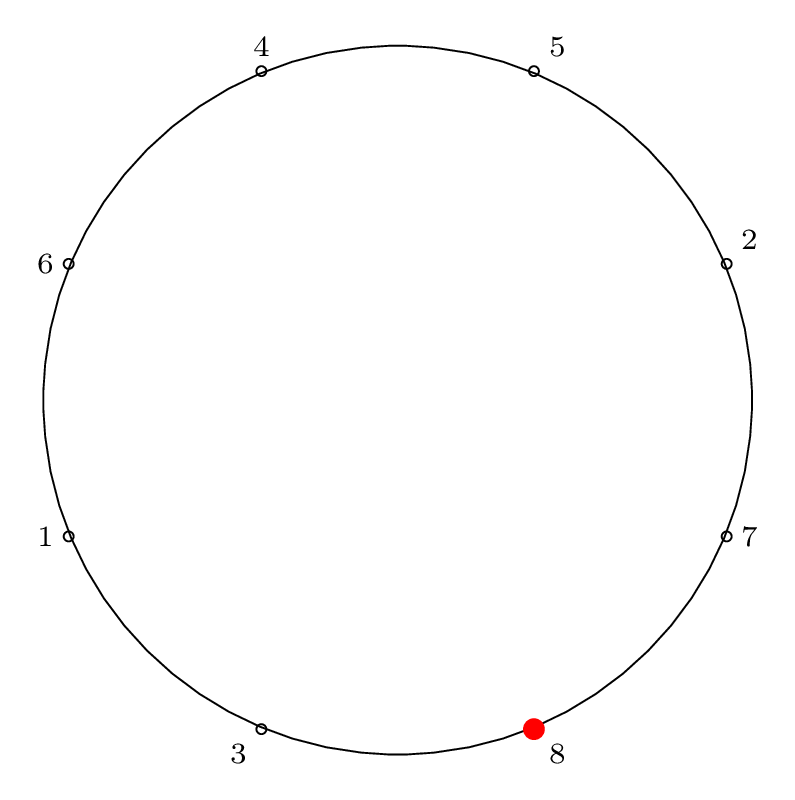### Writing the Solution Permutation: Two Row Notation

As mentioned in Part 1, we can think about the final removal order of sushi plates (or mice, or soldiers) as a special permutation, the Josephus permutation. If we want to write the Josephus permutation using the standard two-row notation for permutations, we would write the circle order (the index of items around the circle) on the top row, and the removal order (the index of items as they are removed) on the bottom row. This gives:

$$\bigl(\begin{smallmatrix} 1 & 2 & 3 & 4 & 5 & 6 & 7 & 8 \\ 5 & 4 & 6 & 1 & 3 & 8 & 7 & 2 \end{smallmatrix}\bigr)$$

While this notation is more intuitive (makes it easier to answer a variety of questions), it is also inconvenient notation, since there are many equivalent ways of writing a single permutation.

### Writing the Solution Permutation: Cycle Notation

In Part 1 we also introduced cycles and cycle notation. Writing a permutation as a cycle is a unique representation of that permutation. By tracing which elements are permuted with other elements, we can turn the two-row representation into a cycle representation.

Here is the Josephus permutation for $$n = 8, m = 4$$ in cycle notation:

$$\left( 1 \, 5 \, 3 \, 6 \, 8 \, 2 \, 4 \right) \left( 7 \right)$$

### Visual Representation of Solution Permutation

To represent the Josephus permutation graphically, we draw lines connecting the edges that permute and form cycles: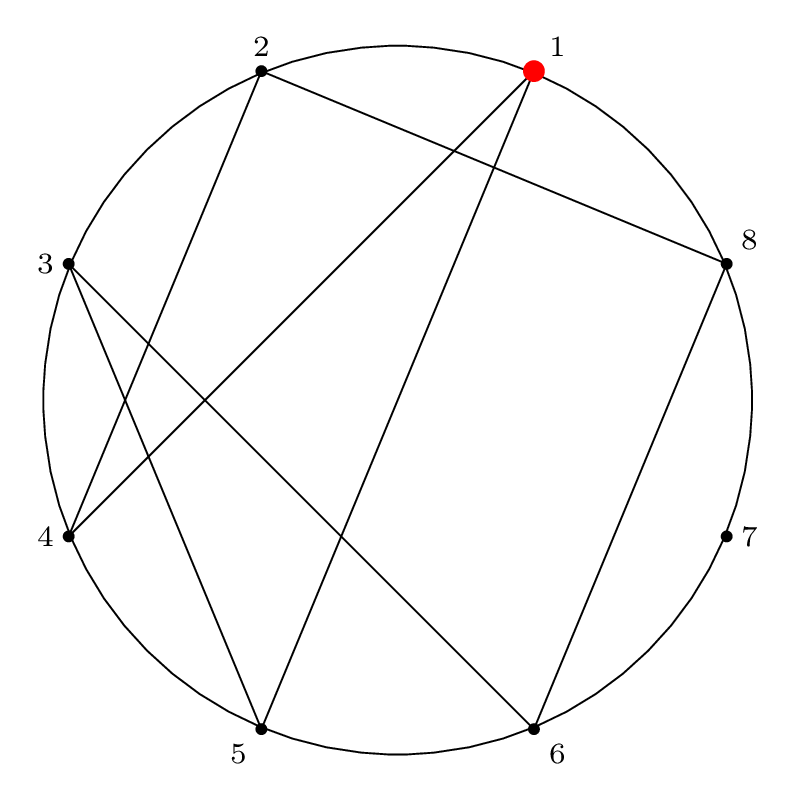This is a visual representation of the Josephus permutation for $$n = 8, m = 4$$.

## n = 11, m = 2

Next is an example with a step size of two, which is a special case of the Josephus problem with a slightly easier solution procedure.

Here is the final Josephus permutation on a circle, which is what our procedure below will yield:

 Circle index: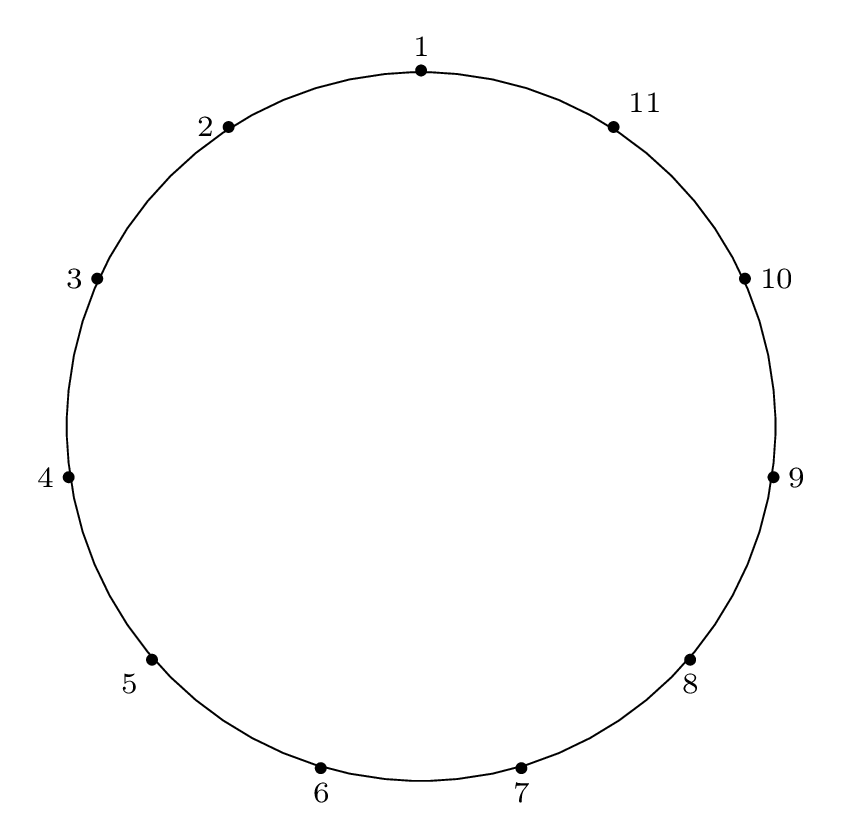Removal index: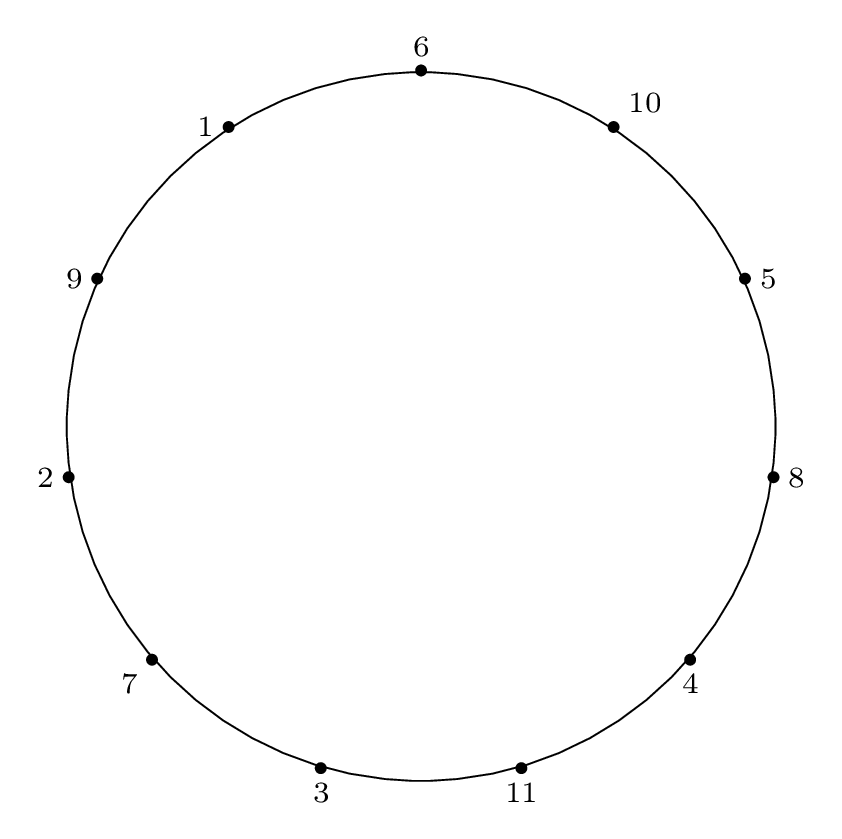### Step by Step Removal for n = 11

As before, we proceed from the red dot, moving forward $$m-1$$ items and removing the $$m^{th}$$ item.

We indicate the starting point with a red dot in Step 0, then indicate the removal index with a red dot and the corresponding removal index.

 Step 0: Starting Point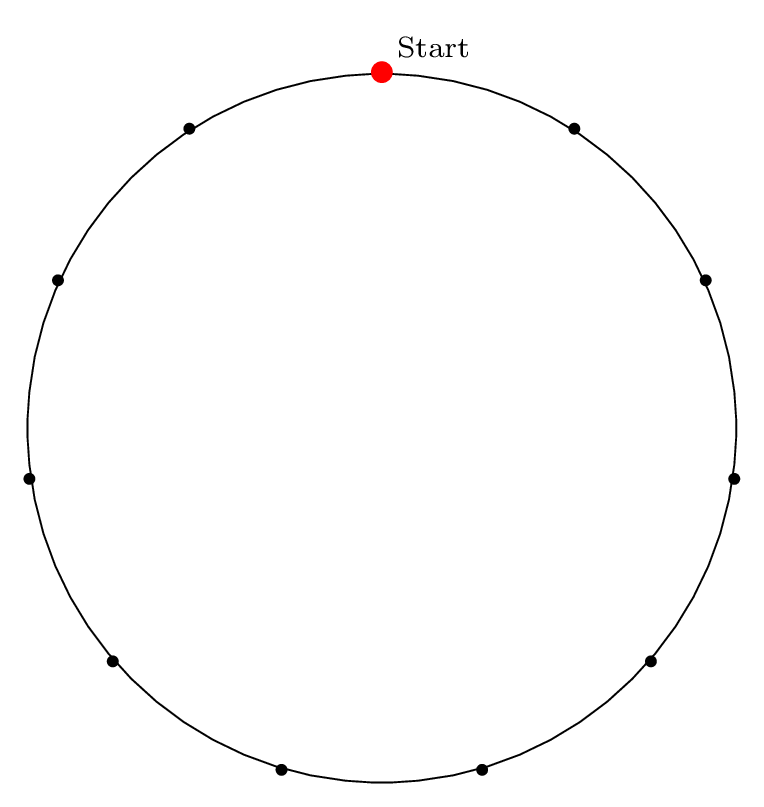Step 1: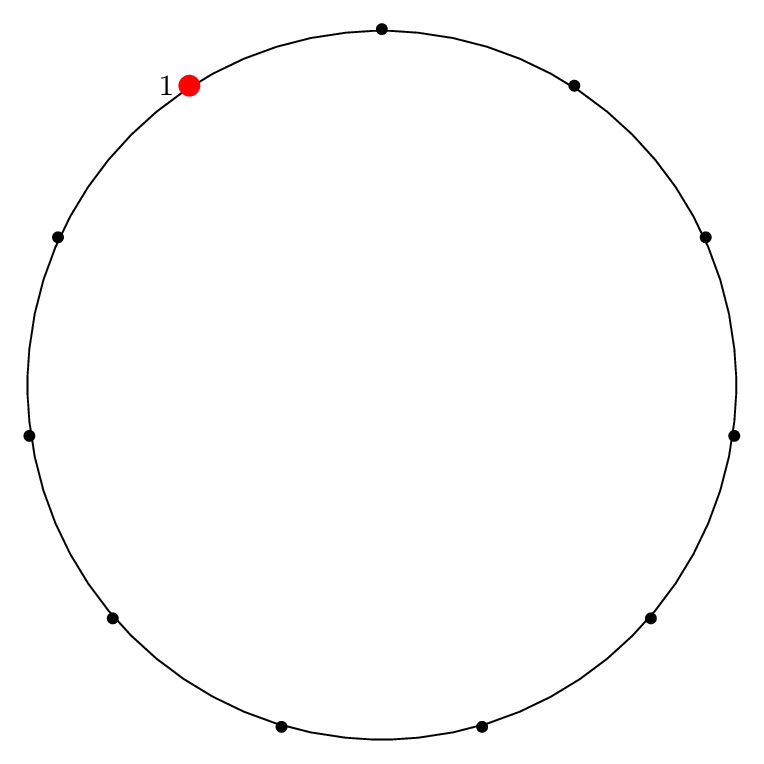Step 2: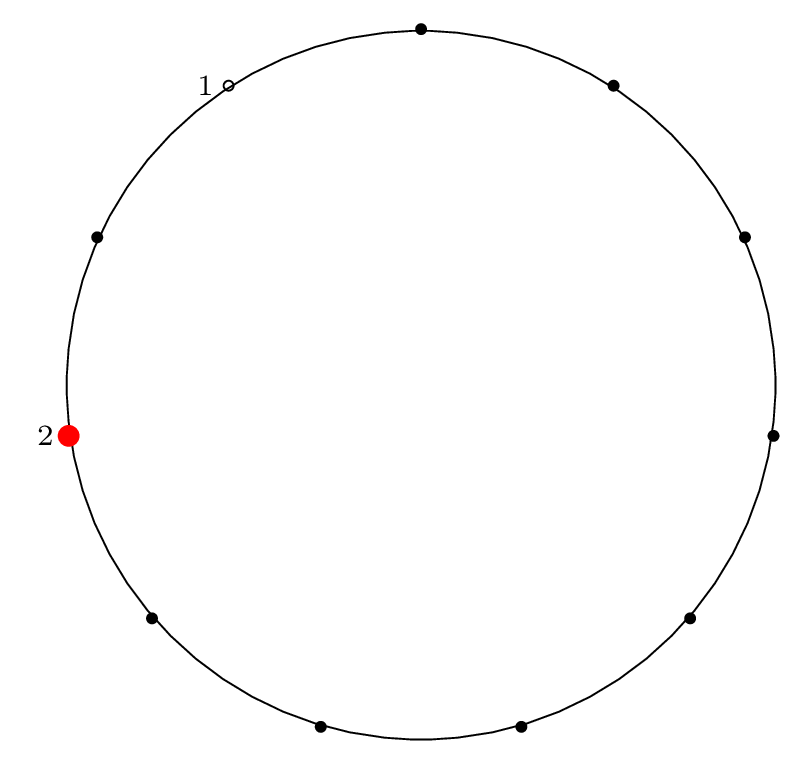Step 3:Step 4: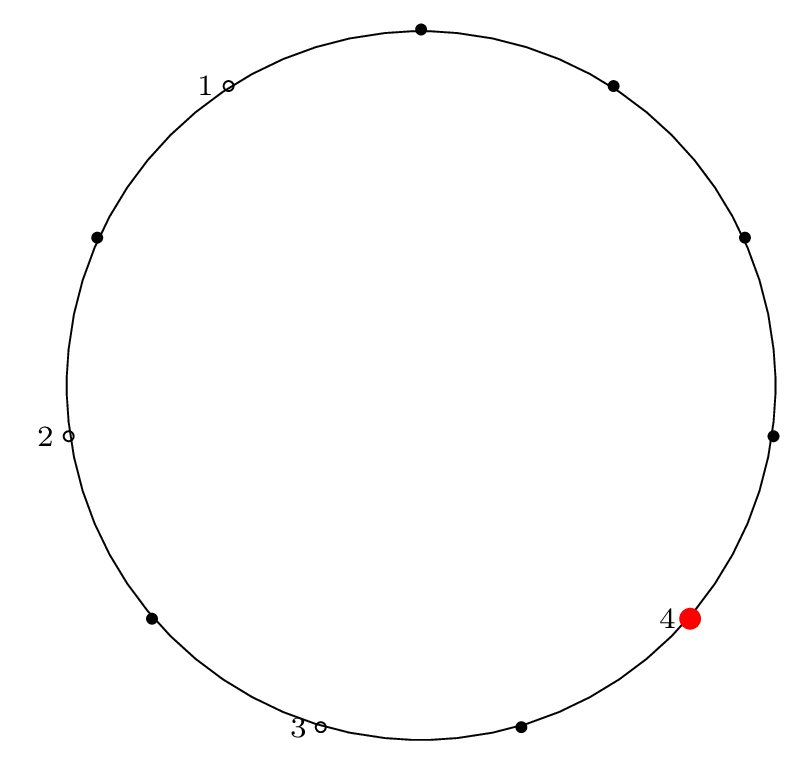Step 5: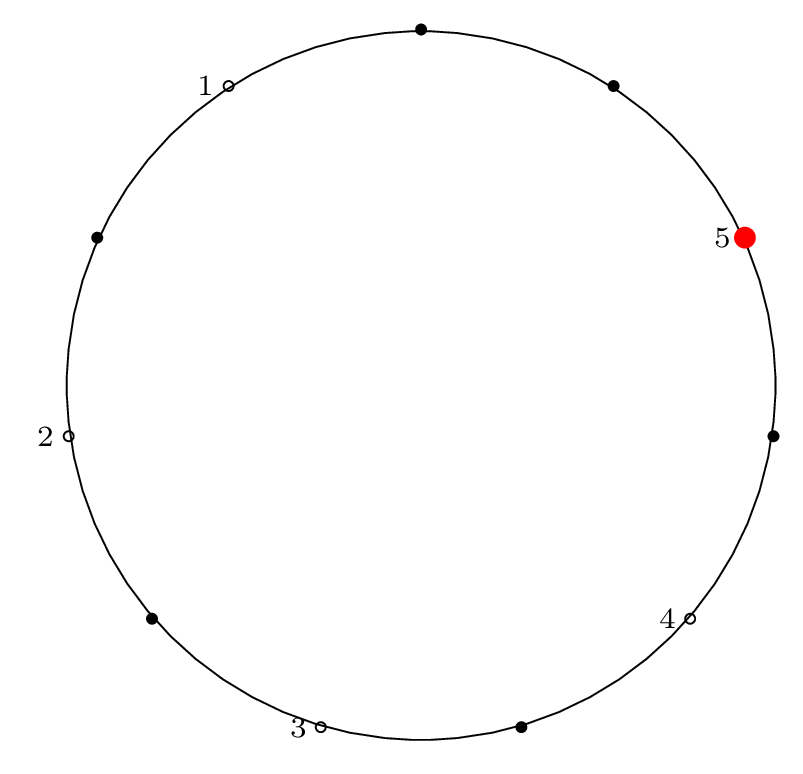Step 6: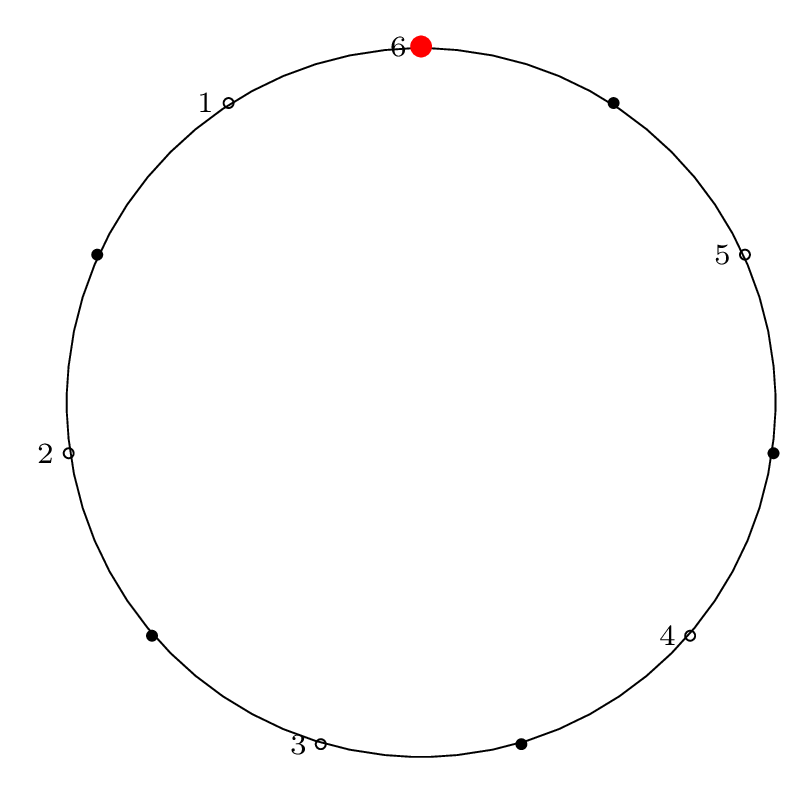Step 7: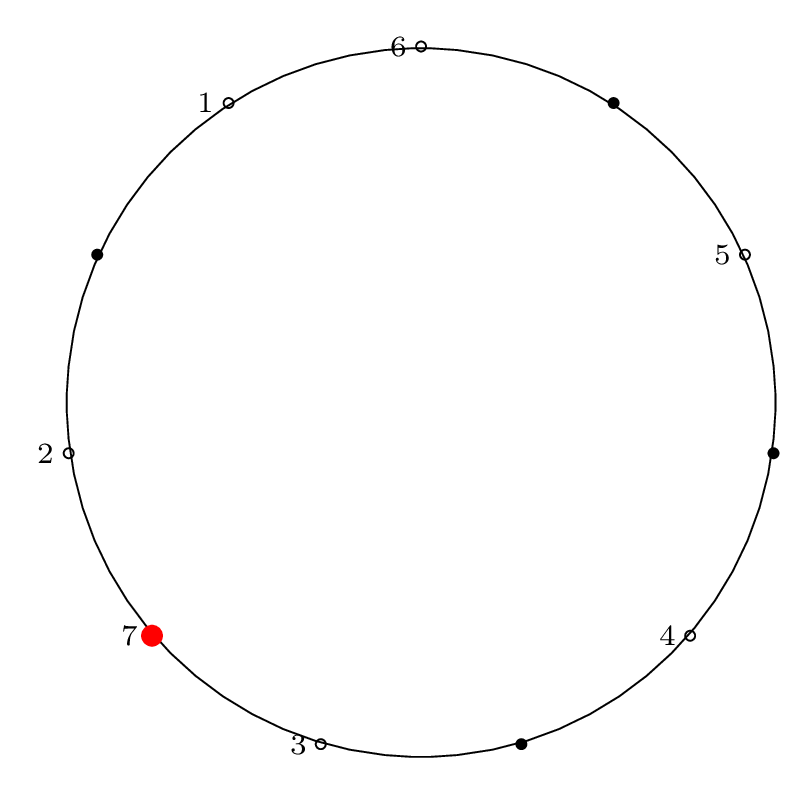Step 8: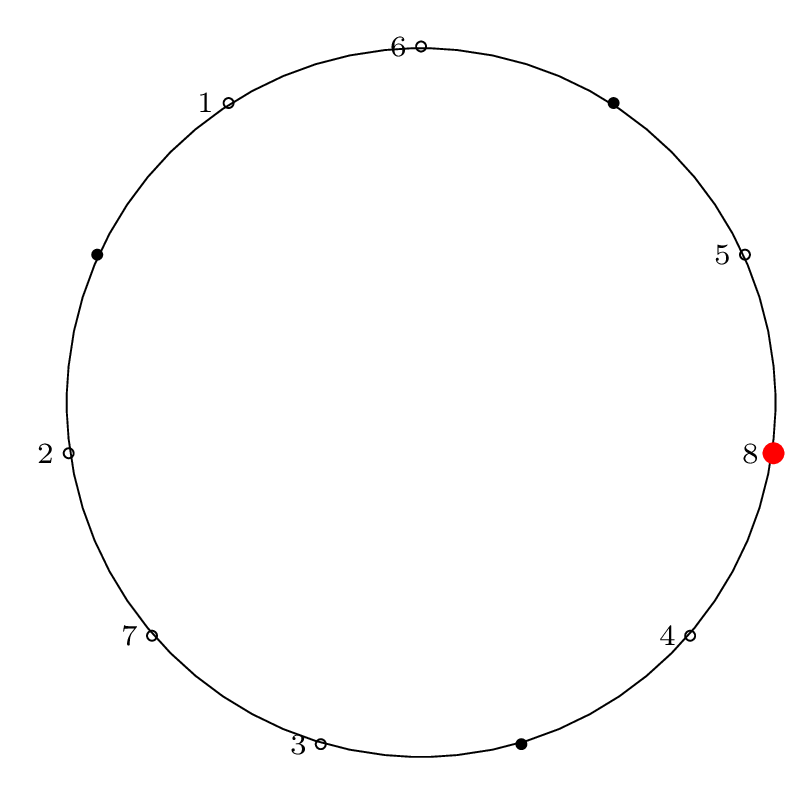Step 9: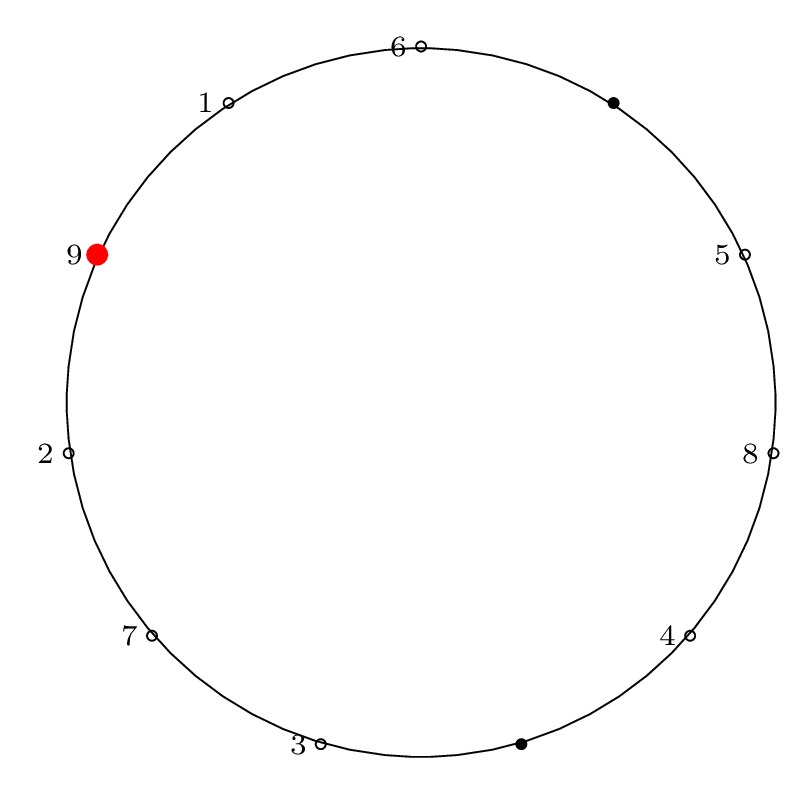Step 10: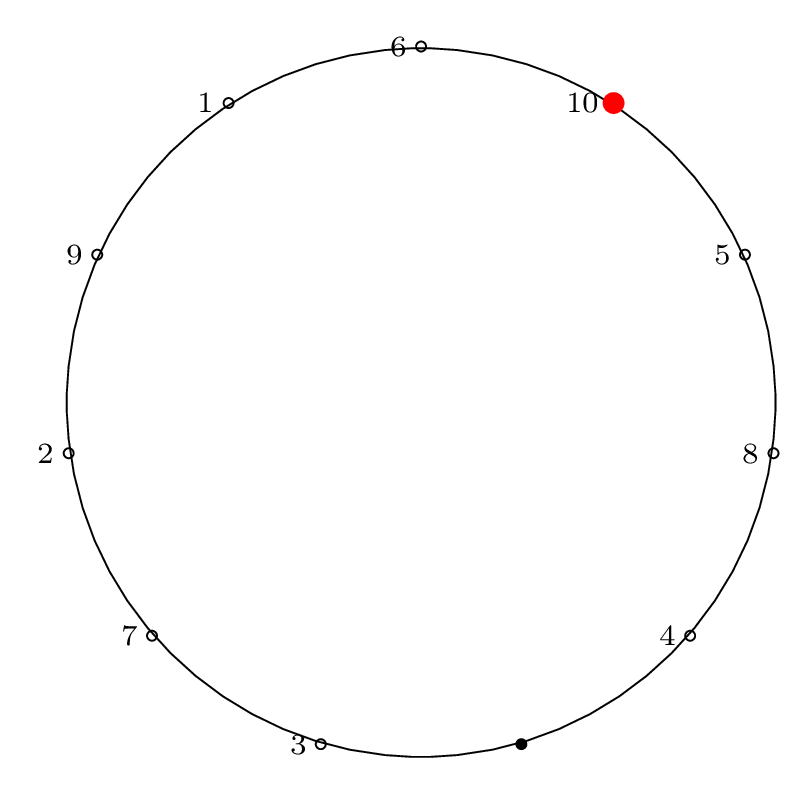Step 11: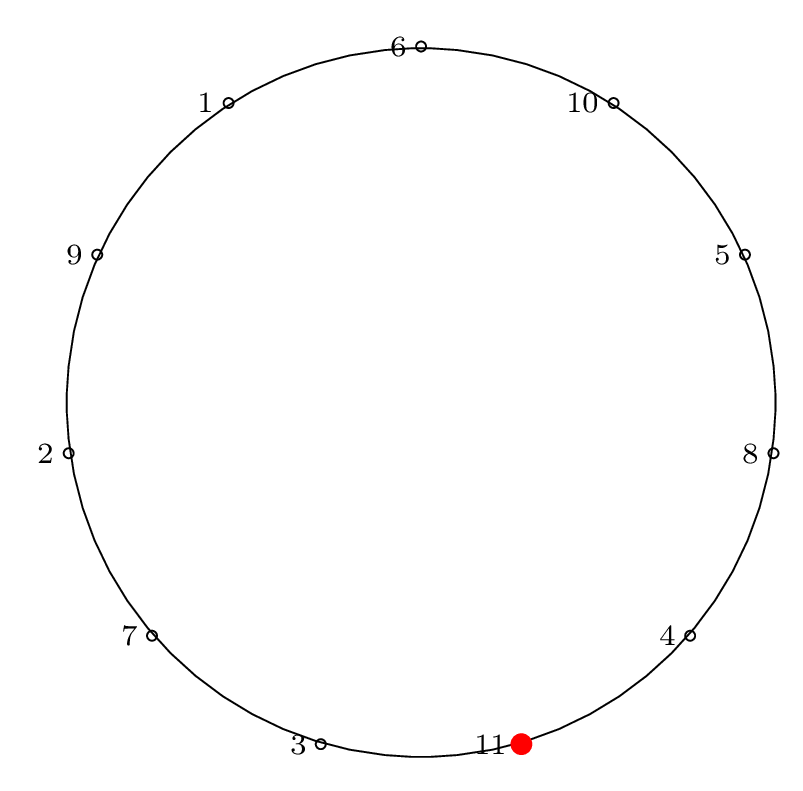### Two Row Notation

Here is the two-row representation:

$$\bigl(\begin{smallmatrix} 1 & 2 & 3 & 4 & 5 & 6 & 7 & 8 & 9 & 10 & 11 \\ 6 & 1 & 9 & 2 & 7 & 3 & 11 & 4 & 8 & 5 & 10 \end{smallmatrix}\bigr)$$

### Cycle Notation

When factored into a cycle, this gives:

$$\left( 1 \, 6 \, 3 \, 9 \, 8 \, 4 \, 2 \right) \left( 5 \, 7 \, 11 \, 10 \right)$$

### Circle Permutation Diagram

To represent the Josephus permutation graphically, we draw lines connecting the edges that permute and form cycles: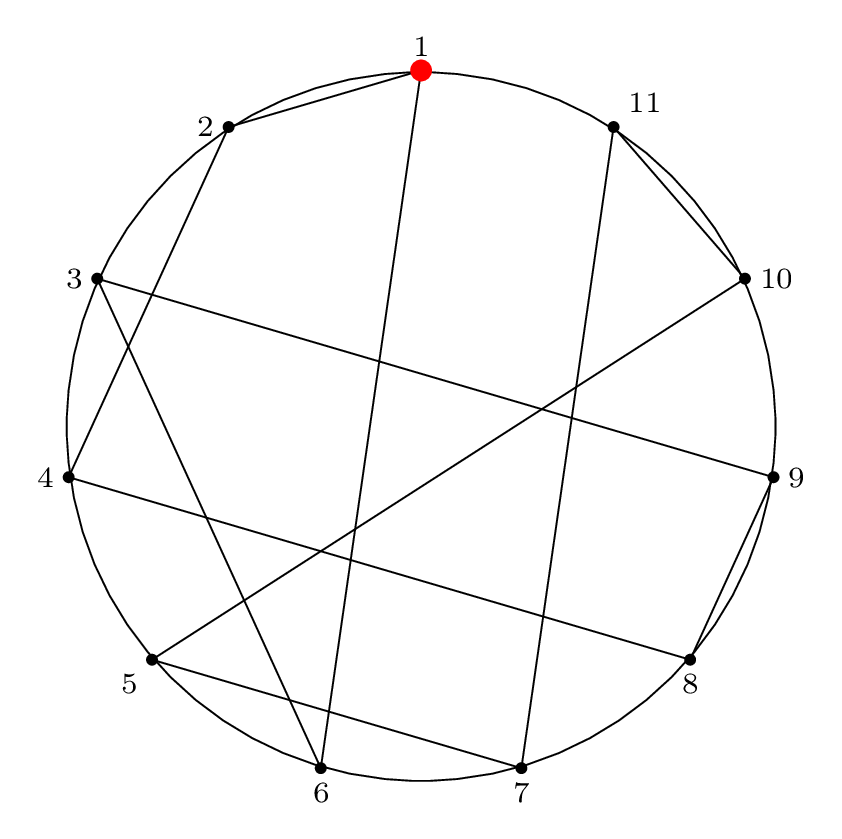This is a visual representation of the Josephus permutation for $$n = 11, m = 2$$.

## Next Steps: Solve!

Our next steps are simple: solve it!

In Part 3 we will show the solution of the special case of $$m = 2$$ (the double-step case).

In Part 4 we will show several ways to solve the general case, and walk through some examples where we apply the solution procedure.

# The Josephus Problem: Part 1: The Problem

Posted in Computer Science

This is Part 1 of an N-part series.

## The Josephus Problem and Variations

The following problem, Cat and Mice, is Puzzle 88 in Boris Kordemsky's The Moscow Puzzles.

Purrer has decided to take a nap. He dreams he is encircled by $$n$$ mice: $$n-1$$ gray and 1 white. He hears is owner saying, "Purrer, you are to eat each $$m$$-th mouse, keeping the same direction. The last mouse you eat must be the white one.

This problem presents a scenario that is nearly identical to a classic problem that has a more grisly backstory: the Josephus problem.

Donald Knuth presents a more unsettling version of this problem, so I'll just let his (rather gleeful) description of the original problem suffice (except - what's this "we" stuff?)

From Section 1.3.2 of The Art of Computer Programming (Vol. 1), Exercise 22 presents the Josephus Problem:

22. (The Josephus Problem.) There are $$n$$ men arranged in a circle. Beginning with a particular position, we count around the circle and brutally execute every $$m^{th}$$ man (the circle closing as men are decapitated). For example, the execution order when $$n = 8, m = 4$$ is $$54613872$$: the first man is fifth to go, the second man is fourth, etc. Write a program which prints outs the order of execution when $$n = 24, m = 11$$. Try to design a clever algorithm that works at high speed when $$n$$ and $$m$$ are large.

### The Sushi Boat Variation

You are at the sushi boat restaurant, where plates of sushi in tiny boats float by in front of you.

There are $$n$$ plates of sushi, each on a sushi boat. Each plate of sushi is labeled $$1 \dots n$$ and arranged in order on the boats.

Beginning at plate 1, you count $$m$$ plates of sushi, stopping at the $$m^{th}$$ boat and taking the plate of sushi off the boat to eat it.

In what order will the sushi plates be stacked when you are done?

Which plate of sushi will be eaten last?

### More Backstory

More background on the Josephus problem and its various solutions is given in a letter to the editor from the Fibonacci Quarterly, Issue 1 of 1976 (Part 1 and Part 2) written in response to an article that gave a solution to the problem of "Idiot's Roulette" (identical to the Josephus problem as presented above) without referencing the Josephus problem. Here is the original article.

## The Tools

To solve the Josephus problem, we need to use several conceptual and computational tools. Below we cover some notation we will use and give links to pages on the charlesreid1.com wiki that are useful.

### Permutations

We can think of the outcome of the Josephus problem as a "Josephus permutation" - a permutation that reorders the sushi plates in the circle (numbered by their positoin in the circle) into the order in which they are removed from the circle.

For example, in Knuth's problem statement, he gives an example of $$n = 8, m = 4$$ (eating every 4th plate of sushi, in a train of 8 sushi boats), which results in the plates being removed in the following order:

$$5, 4, 6, 1, 3, 8, 7, 2$$

To write this permutation using mathematical notation, we write two rows. The top row is the ordering of plates in the circle, the "natural" ordering, and the second row is the order of removal of plates, which is the Josephus permutation:

$$\bigl(\begin{smallmatrix} 1 & 2 & 3 & 4 & 5 & 6 & 7 & 8 \\ 5 & 4 & 6 & 1 & 3 & 8 & 7 & 2 \end{smallmatrix}\bigr)$$

As Knuth would point out, this Josephus permutation can be written in 40,319 other equivalent ways (that's 8! total, minus the 1 way shown above) by reordering the columns (as long as we reorder the columns in the top and bottom rows in the same way).

We can read this permutation from left to right as follows:

• The first sushi plate (labeled 1) will be eaten fifth;
• The second sushi plate (labeled 2) will be eaten fourth;
• etc.

Ordering the permutation as above (circle index on top, removal index on bottom) makes it easy to answer the second question, "Which sushi plate will be eaten last?"

We find 8 in the bottom row (the removal index), and read the corresponding number in the top row (the plate number/circle position), plate 6.

If we wish to answer the first question, "in what order will the plates be removed," we have a bit more work to do. We mentioned that the above permutation is 1 of a total of 40,320 equivalent ways of writing the same permutation. Another way of writing it would be to maintain the pairing between top and bottom but sort the bottom elements:

$$\bigl(\begin{smallmatrix} 4 & 8 & 5 & 2 & 1 & 3 & 7 & 6 \\ 1 & 2 & 3 & 4 & 5 & 6 & 7 & 8 \end{smallmatrix}\bigr)$$

Now we can find the order of the plates by reading the top row right-to-left. The last plate removed is plate 6, so that will be on top of the stack of plates (stacks are first in, last out).

We cover permutations and permutation notation in the context of Rubiks Cubes on the Rubiks Cube/Permutations page of the charlesreid1.com wiki.

### Cycles

While the above permutation notation is useful, the variety of ways of expressing the same permutation is inconvenient. This is where cycles become useful - cycles are a way of implicitly representing both rows of the permutation.

To do this, we "thread" our way through the permutation to create the cycle of which items move to which positions.

Starting with the left-most column of the permutation,

$$\bigl(\begin{smallmatrix} 1 & 2 & 3 & 4 & 5 & 6 & 7 & 8 \\ 5 & 4 & 6 & 1 & 3 & 8 & 7 & 2 \end{smallmatrix}\bigr)$$

we know that $$1 \rightarrow 5$$. Now we find the column that has 5 in the top: the fifth column. We write $$5 \rightarrow 3$$. Now we find the column with 3 in the top: the third column. We write $$3 \rightarrow 6$$. Next, we write $$6 \rightarrow 8$$, then $$8 \rightarrow 2$$, then $$2 \rightarrow 4$$. Once we see that the last step of the cycle is $$4 \rightarrow 1$$, which brings us back to the beginning, we write that closed cycle in parentheses. If we have elements left, we repeat the procedure starting with any of the remaining elements. Sometimes there is a single cycle, and sometimes there are multiple cycles. In this case we have two cycles:

$$\left( 1 \, 5 \, 3 \, 6 \, 8 \, 2 \, 4 \right) \left( 7 \right)$$

This indicates that 7 does not change position, i.e., the 7th plate of sushi is eaten 7th.

This notation is very convenient for finding solutions, and it turns out that Knuth gives a general solution procedure for the Josephus problem that involves a rather complicated application of the cycle notation, so a solid understanding of cycle notation is important.

We cover cycle notation in the context of Rubiks Cubes on the Rubiks Cube/Permutations page of the charlesreid1.com wiki (in particular, the sections on Permutation Algebra that cover intercalation products).

### Circular Linked Lists

Among the many ways of solving the Josephus problem, the easiest method is to just carry out the procedure by hand to find the final Josephus permutation, then use it to answer the original question. This technique is referred to as the simulation technique.

This technique would become infeasible if we were to ask more difficult versions of the Josephus problem, such as posing a scenario where there are 5 million plates of sushi, and we wish to know which position (which plate of sushi) will be eaten 2,999,998th, and we also wish to have the answer instantaneously.

If we're dealing with smaller values of n and m, though, we can simulate a solution to the Josephus problem using a circular linked list.

Briefly, a linked list is a type of list whose elements consist of nodes, small bundles containing a piece of data (the list item's value) and pointers to other nodes (the next and/or previous elements in the list).

See Linked Lists for notes on linked lists and some answers to textbook exercises.

See Lists Study Guide for a summary of important information about and properties of lists.

A circular linked list is just what it sounds like: each of the elements points to the next element, and the last element in the list points to the first element in the list. We can use the list by maintaining a pointer to a particular item (the first item in the list).

We can insert items into the list by creating a new node, and inserting it between two nodes (e.g., the front and back of the list) by updating the pointers of the front and back nodes to point to the new list item.

Usefully, we can also remove items from the list with some pointer manipulation. To remove a node, we modify the next/previous pointers of the nodes before/after the doomed node so that they point to each other instead of to the doomed node.

This allows us to explicitly model the sushi boat (a.k.a. the "kill ring") in the Josephus problem.

Circular linked lists are covered on the charlesreid1.com wiki on the Linked Lists/Java/Circular page, and are implemented in Java in the cs/java repo: https://git.charlesreid1.com/cs/java/src/branch/master/lists/linked-lists

A Python implementation used to solve the Josephus problem is available in the cs/josephus repo: repo: https://git.charlesreid1.com/cs/josephus

## TeX for Diagrams

In addition to writing The Art of Computer Programming, which has remained a gold standard algorithm textbook for over 40 years, Knuth also invented the TeX typesetting system, which is also the gold standard for typesetting mathematical equations.

We use the PGF/TikZ package to draw polygons that are useful in illustrating the circles of the Josephus problem and in visualizing various permutations.

A few examples and links to Github Gists with TeX code follow.

### Empty Josephus Circle Diagram

Link to gist with TeX codeHere is the TeX code to generate this diagram:

\documentclass[border=2mm]{standalone}
\usepackage{tikz}
\usepackage{xintexpr}
\usetikzlibrary{shapes.geometric}

\begin{document}
\begin{tikzpicture}[scale=3]

% make a node with variable name pol (with the list of features given) at the location (0,0), and don't label it
\node (pol) [draw=none, thick, black!90!black,rotate=0,minimum size=6cm,regular polygon, regular polygon sides=11] at (0,0) {};

% anchor is "corner 1"
% label is 1/2/3/4/etc
% placement is placement w.r.t. coordinate location
\foreach \anchor/\label/\placement in
{corner 1/$1$/above,
corner 2/$2$/above left,
corner 3/$3$/left,
corner 4/$4$/left,
corner 5/$5$/below left,
corner 6/$6$/below,
corner 7/$7$/below,
corner 8/$8$/below right,
corner 9/$9$/right,
corner 10/${10}$/right,
corner 11/${11}$/above right}
\draw[shift=(pol.\anchor)] plot coordinates{(0,0)} node[font=\scriptsize,\placement] {\label};

% draw a circle connecting all points

% Draw a red dot at the starting point
\filldraw[red] (pol.corner 1) circle[radius=0.8pt];

% optional: black dots at each circle location
\filldraw[black] (pol.corner 2) circle[radius=0.4pt];
\filldraw[black] (pol.corner 3) circle[radius=0.4pt];
\filldraw[black] (pol.corner 4) circle[radius=0.4pt];
\filldraw[black] (pol.corner 5) circle[radius=0.4pt];
\filldraw[black] (pol.corner 6) circle[radius=0.4pt];
\filldraw[black] (pol.corner 7) circle[radius=0.4pt];
\filldraw[black] (pol.corner 8) circle[radius=0.4pt];
\filldraw[black] (pol.corner 9) circle[radius=0.4pt];
\filldraw[black] (pol.corner 10) circle[radius=0.4pt];
\filldraw[black] (pol.corner 11) circle[radius=0.4pt];

\end{tikzpicture}
\end{document}


### Josephus Circle Diagram With Permutation Paths

Next, we can illustrate cycles in the permutation by drawing paths between connected nodes.

The edges are directed (1 -> 4 is not the same as 4 -> 1). We draw both directed and undirected versions.

Link to gist with TeX code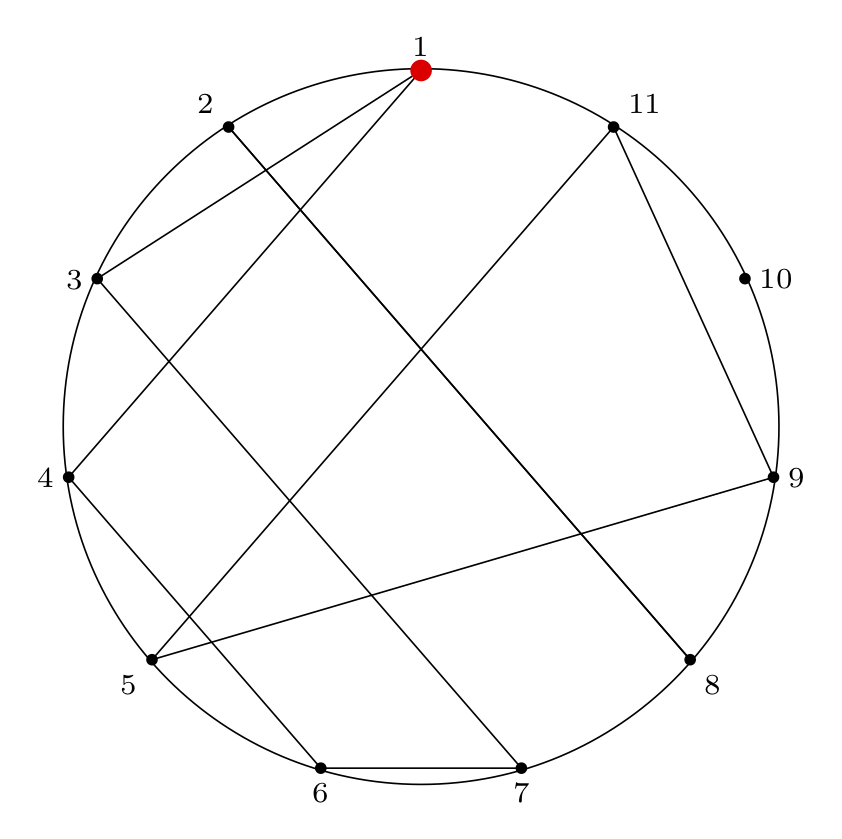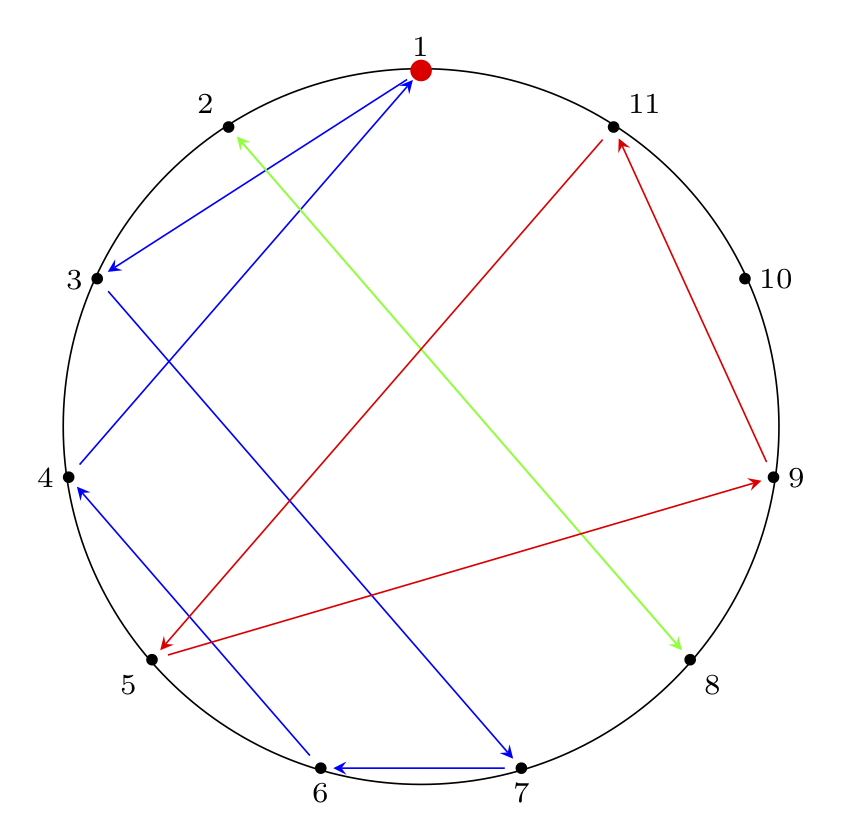The code to generate these diagrams is below.

Undirected Paths:

\documentclass[border=2mm]{standalone}
\usepackage{tikz}
\usepackage{xintexpr}
\usetikzlibrary{shapes.geometric}

\begin{document}
\begin{tikzpicture}[scale=3]

% make a node with variable name pol (with the list of features given) at the location (0,0), and don't label it
\node (pol) [draw=none, thick, black!90!black,rotate=0,minimum size=6cm,regular polygon, regular polygon sides=11] at (0,0) {};

% anchor is "corner 1"
% label is 1/2/3/4/etc
% placement is placement w.r.t. coordinate location
\foreach \anchor/\label/\placement in
{corner 1/$1$/above,
corner 2/$2$/above left,
corner 3/$3$/left,
corner 4/$4$/left,
corner 5/$5$/below left,
corner 6/$6$/below,
corner 7/$7$/below,
corner 8/$8$/below right,
corner 9/$9$/right,
corner 10/${10}$/right,
corner 11/${11}$/above right}
\draw[shift=(pol.\anchor)] plot coordinates{(0,0)} node[font=\scriptsize,\placement] {\label};

% solution for n = 11, m = 4:
% ( 1 3 7 6 4 ) ( 2 8 ) ( 5 9 11 ) ( 10 )

% internal paths

% cycle (1 3 7 6 4)
\path [-] (pol.corner 1) edge (pol.corner 3);
\path [-] (pol.corner 3) edge (pol.corner 7);
\path [-] (pol.corner 7) edge (pol.corner 6);
\path [-] (pol.corner 6) edge (pol.corner 4);
\path [-] (pol.corner 4) edge (pol.corner 1);

% cycle 2 (2 8)
\path [-] (pol.corner 2) edge (pol.corner 8);
\path [-] (pol.corner 8) edge (pol.corner 2);

% cycle 3 (5 9 11 )
\path [-] (pol.corner 5) edge (pol.corner 9);
\path [-] (pol.corner 9) edge (pol.corner 11);
\path [-] (pol.corner 11) edge (pol.corner 5);

% draw a circle connecting all points

% Draw a red dot at the starting point
\filldraw[red] (pol.corner 1) circle[radius=0.8pt];

% optional: black dots at each circle location
\filldraw[black] (pol.corner 2) circle[radius=0.4pt];
\filldraw[black] (pol.corner 3) circle[radius=0.4pt];
\filldraw[black] (pol.corner 4) circle[radius=0.4pt];
\filldraw[black] (pol.corner 5) circle[radius=0.4pt];
\filldraw[black] (pol.corner 6) circle[radius=0.4pt];
\filldraw[black] (pol.corner 7) circle[radius=0.4pt];
\filldraw[black] (pol.corner 8) circle[radius=0.4pt];
\filldraw[black] (pol.corner 9) circle[radius=0.4pt];
\filldraw[black] (pol.corner 10) circle[radius=0.4pt];
\filldraw[black] (pol.corner 11) circle[radius=0.4pt];

\end{tikzpicture}
\end{document}


Directed Paths:

\documentclass[border=2mm]{standalone}
\usepackage{tikz}
\usepackage{xintexpr}
\usetikzlibrary{shapes.geometric}

\begin{document}
\begin{tikzpicture}[scale=3]

% make a node with variable name pol (with the list of features given) at the location (0,0), and don't label it
\node (pol) [draw=none, thick, black!90!black,rotate=0,minimum size=6cm,regular polygon, regular polygon sides=11] at (0,0) {};

% anchor is "corner 1"
% label is 1/2/3/4/etc
% placement is placement w.r.t. coordinate location
\foreach \anchor/\label/\placement in
{corner 1/$1$/above,
corner 2/$2$/above left,
corner 3/$3$/left,
corner 4/$4$/left,
corner 5/$5$/below left,
corner 6/$6$/below,
corner 7/$7$/below,
corner 8/$8$/below right,
corner 9/$9$/right,
corner 10/${10}$/right,
corner 11/${11}$/above right}
\draw[shift=(pol.\anchor)] plot coordinates{(0,0)} node[font=\scriptsize,\placement] {\label};

% solution for n = 11, m = 4:
% ( 1 3 7 6 4 ) ( 2 8 ) ( 5 9 11 ) ( 10 )

% internal paths

% cycle (1 3 7 6 4)
\path [->, shorten > = 3 pt, blue, shorten < = 4 pt, > = stealth] (pol.corner 1) edge (pol.corner 3);
\path [->, shorten > = 3 pt, blue, shorten < = 4 pt, > = stealth] (pol.corner 3) edge (pol.corner 7);
\path [->, shorten > = 3 pt, blue, shorten < = 4 pt, > = stealth] (pol.corner 7) edge (pol.corner 6);
\path [->, shorten > = 3 pt, blue, shorten < = 4 pt, > = stealth] (pol.corner 6) edge (pol.corner 4);
\path [->, shorten > = 3 pt, blue, shorten < = 4 pt, > = stealth] (pol.corner 4) edge (pol.corner 1);

% cycle 2 (2 8)
\path [->, shorten > = 3 pt, green, shorten < = 4 pt, > = stealth] (pol.corner 2) edge (pol.corner 8);
\path [->, shorten > = 3 pt, green, shorten < = 4 pt, > = stealth] (pol.corner 8) edge (pol.corner 2);

% cycle 3 (5 9 11 )
\path [->, shorten > = 3 pt, red, shorten < = 4 pt, > = stealth] (pol.corner 5) edge (pol.corner 9);
\path [->, shorten > = 3 pt, red, shorten < = 4 pt, > = stealth] (pol.corner 9) edge (pol.corner 11);
\path [->, shorten > = 3 pt, red, shorten < = 4 pt, > = stealth] (pol.corner 11) edge (pol.corner 5);

% draw a circle connecting all points

% draw a red dot at the starting point
\filldraw[red] (pol.corner 1) circle[radius=0.8pt];

% optional: black dots at each circle location
\filldraw[black] (pol.corner 2) circle[radius=0.4pt];
\filldraw[black] (pol.corner 3) circle[radius=0.4pt];
\filldraw[black] (pol.corner 4) circle[radius=0.4pt];
\filldraw[black] (pol.corner 5) circle[radius=0.4pt];
\filldraw[black] (pol.corner 6) circle[radius=0.4pt];
\filldraw[black] (pol.corner 7) circle[radius=0.4pt];
\filldraw[black] (pol.corner 8) circle[radius=0.4pt];
\filldraw[black] (pol.corner 9) circle[radius=0.4pt];
\filldraw[black] (pol.corner 10) circle[radius=0.4pt];
\filldraw[black] (pol.corner 11) circle[radius=0.4pt];

\end{tikzpicture}
\end{document}


## Next Steps: Examples and Solutions

So far in Part 1 we have covered some common forms of the Josephus problem.

In Part 2 we'll cover some examples of different $$n, m$$ values ($$n$$ is circle size, $$m$$ is skip length) and show how the process plays out.

In Part 3 we will show the solution of the special case of $$m = 2$$ (the double-step case).

In Part 4 we will show several ways to solve the general case, and walk through some examples where we apply the solution procedure.